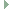OGRE  13.4 Object-Oriented Graphics Rendering Engine
Ogre::LinearControllerFunction Class Reference

Predefined controller function based on linear function interpolation. More...

#include <OgrePredefinedControllers.h>Inheritance diagram for Ogre::LinearControllerFunction:

## Public Member Functions

LinearControllerFunction (const std::vector< Real > &keys, const std::vector< Real > &values, Real frequency=1, bool deltaInput=true)

Real calculate (Real source)Public Member Functions inherited from Ogre::ControllerFunction< Real >
ControllerFunction (bool deltaInput)
Constructor. More...

virtual ~ControllerFunction ()

## Static Public Member Functions

static ControllerFunctionRealPtr create (const std::vector< Real > &keys, const std::vector< Real > &values, Real frequency=1, bool deltaInput=true)
Constructor, requires keys and values of the function to interpolate. More...

## Detailed Description

Predefined controller function based on linear function interpolation.

## ◆ LinearControllerFunction()

 Ogre::LinearControllerFunction::LinearControllerFunction ( const std::vector< Real > & keys, const std::vector< Real > & values, Real frequency = 1, bool deltaInput = true )

## ◆ create()

 static ControllerFunctionRealPtr Ogre::LinearControllerFunction::create ( const std::vector< Real > & keys, const std::vector< Real > & values, Real frequency = 1, bool deltaInput = true )
inlinestatic

Constructor, requires keys and values of the function to interpolate.

For simplicity and compatibility with the predefined ControllerValue classes the function domain must be [0,1]. However, you can use the frequency parameter to rescale the domain to a different range.

Parameters
 keys the x-values of the function sampling points. Value range is [0,1]. Must include at least the keys 0 and 1. values the function values f(x) of the function. order must match keys frequency the speed of the evaluation in cycles per second deltaInput If true, signifies that the input will be a delta value such that the function should add it to an internal counter before calculating the output.
Note
there must be the same amount of keys and values

## ◆ calculate()

 Real Ogre::LinearControllerFunction::calculate ( Real source )
virtual

The documentation for this class was generated from the following file: